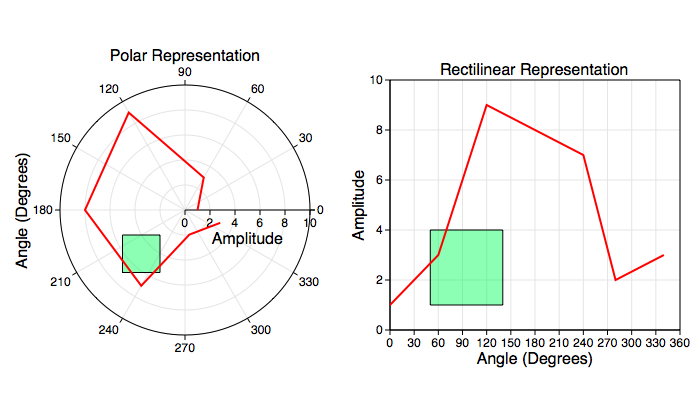Graph IDEGraphs ► Non-Linear Graphs

Non-Linear Graphs are graphs whose dimensions are not represented by uniformly spaced straight lines. For example, the polar graph shown here:is considered non-linear while the rectilinear graph on the right is not. There are two graphics on each of those graphs, a green rectangle and a red sequence of line segments (a curve). The curve points are described in the circular and radial grid coordinate lines (namely {θ, r}) while the rectangle, which is a graphic-oriented graphic, is described in mapped rectilinear coordinates (namely {x, y}). The relationship is: r = sqrt(x x + y y) and θ = atan(y/x). The {θ, r} coordinate is called the domain space, while the {x, y} coordinate is called the range space.

So that the green rectangle has origin at point {-5, -5} and size {3, 3} for the left (polar) graph and origin at point {50, 1} and size {90, 3} for the right (rectilinear) graph. This is in contrast to the points that define the red curve. For both graphs the red curve has the same point values:

 θ (Degrees) Amplitude 0 1 60 3 120 9 180 8 240 7 280 2 340 3

That is because the curve is defined in the domain space of each graph, while the rectangle is defined in the range space of the graph. For general non-linear graphs there are three different representations (spaces) to be aware of:

 Representation Name Description Domain This is the coordinate system as shown by the graph's grid lines and curves. Range This is the coordinate system after the non-linear mapping takes place and is a linear coordinate system. For example: xp = log(x). Rectilinear This is a secondary representation of either the domain or range representation. For polar coordinates this is another representation of the domain space, for log coordinates this is the range space.

The only coordinate system where the three representations described above are unique is the log-r polar graph. The only one where they are the same (and hence only the Domain Representation is considered) is the normal Linear Axis graph.

The important part of non-linear graphs is to keep the representations in your mind while working with the graph, and which representation corresponds to which graphic. When dealing with multiple y-axis or x-axis graphs with linear and nonlinear axis this issue needs to be well thought out because one area on the graph can have many coordinate systems and spaces.